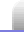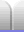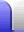# Gravity

Gravity is the attraction between objects due to their mass. The gravitation attraction between two objects is proportional to the product of the mass of the objects (the two masses multiplied by each other). It has unlimited range, and gets weaker with increasing distance according to the square of the distance between two objects.

Gravity is the force that keeps us on the planet, and that keeps Earth orbiting the Sun.

Gravity is always attractive, never repulsive.

### Mass

Mass is a property of objects. It determines how resistant an object is to acceleration by a force. The more mass, the larger the force needed to accelerate the object and the greater its gravitational attraction to other objects is.When someone jumps off a wall they accelerate downward. Newton's Third Law of Motion says that at the same time Earth experiences an upward acceleration from an equal and opposite force. In other words, a falling person and Earth accelerate towald each other. Because Earth has so much more mass than a person, Earth hardly moves at all.

The unit of mass, the kilogram (kg), is one of the basic units of measurement. Mass is difficult to measure directly, so weight is normally measured instead.

### Weight

In everyday life, mass and weight are often used interchangeably, but they are different things.

Weight is the force taken to stop an object (with mass) from accelerating.

The direction "down" depends on gravity or an acceleration. Down is the direction in which we experience weight, or the direction in which we are accelerating. In other words, if we are standing on the ground, our weight tells us which way is down. If we are falling, the direction we are falling is down.

### Freefall

An object in a gravitational field that is not restrained from falling will fall unrestricted. It is said to be in freefall. Objects in freefall are weightless.Home Astronomy Chemistry Electronics & Computers Mathematics Physics Field Trips Turn on javascript for email link